# ACT Science Practice Test 2

Directions: The ACT Science passage below is followed by several questions. After reading the passage, choose the best answer to each question. You may refer to the passage as often as necessary. Calculators may NOT be used on this test.

Passage II

A scientist wishes to study the relationship between the pressure, volume, and temperature of a gas. He conducts three experiments, the results of which are recorded below.

Experiment 1: The scientist varies the volume and pressure of gas in a cylinder while keeping all other factors constant. The scientist records the results in table 1 below.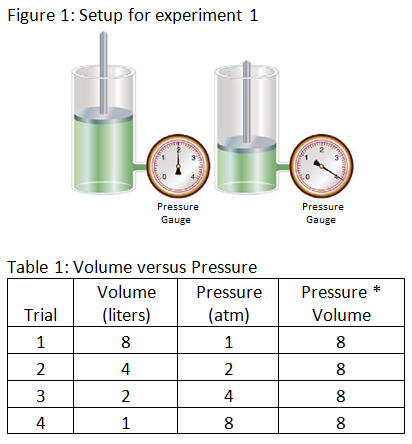Experiment 2: The scientist varies the volume and temperature of gas in a cylinder while keeping all other factors constant. The scientist records the results in table 2 below.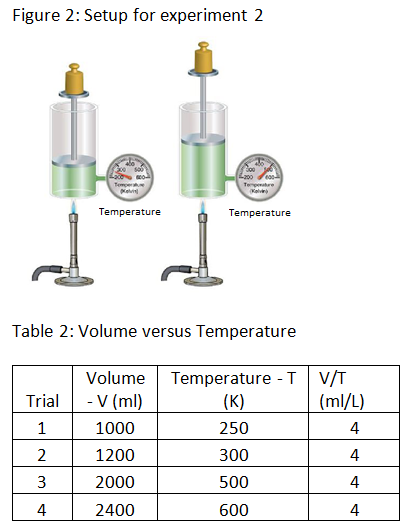Experiment 3: The scientist alters the temperature of gas in a cylinder by heating the cylinder and measures the pressure at each temperature. The scientist records the results in table 3 below.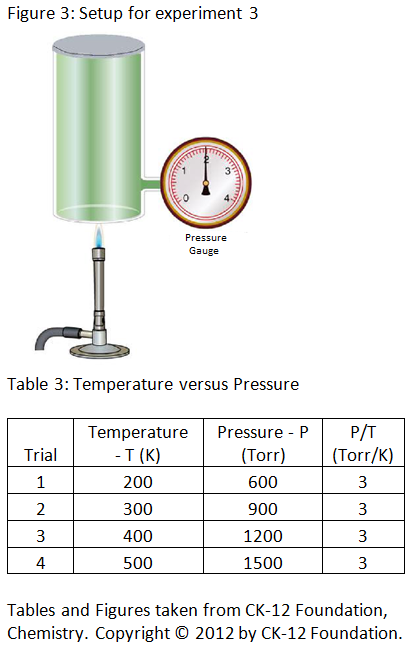Congratulations - you have completed .

You scored %%SCORE%% out of %%TOTAL%%.

Your performance has been rated as %%RATING%%

 Question 1
Based on the results of experiment 3, if the pressure of a gas is doubled and the volume and amount of the gas in the cylinder is held constant, what will be the impact on temperature?

 A It will stay the same. B It will be doubled. C It will be halved. D It will be quadrupled.
Question 1 Explanation:
The correct answer is (B). From table 3, it can be seen that as the temperature increases, the pressure increases. Since temperature is directly proportional to pressure (P/T = 3), it can be inferred that doubling the pressure of the gas will lead to a doubling of its temperature.
 Question 2
Which of the following could be the graph of ‘Pressure × Volume versus Pressure?’

 AB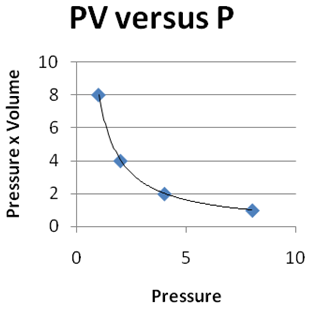C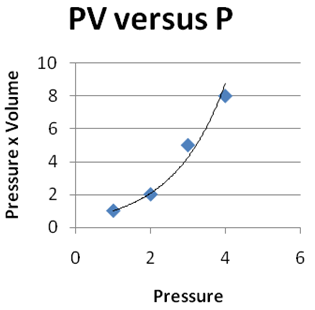DQuestion 2 Explanation:
The correct answer is (D). From table 1, it can be seen that ‘Pressure × Volume’ is constant as pressure increases. Only answer choice (D) shows a constant ‘Pressure × Volume’ as the ‘Pressure’ increases.
 Question 3
Based on the results of experiment 2, what would be the volume of the gas in the cylinder if the cylinder is heated to 350K?

 A 1300 ml B 1400 ml C 1500 ml D 1600 ml
Question 3 Explanation:
The correct answer is (B). From table 2, it can be seen that the ratio of volume to temperature is constant. This constant ratio represents the slope of a linear relationship; volume divided by temperature will always equal 4. The question gives a temperature; the table provides the ratio value, so the unknown volume can be calculated. Stated algebraically: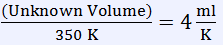Multiply both sides by 350 K to determine the volume (notice that multiplying both sides by units of K yields a quantity in units of milliliters).

Unknown Volume = 1400 ml
 Question 4
Which of the following experiments will best help the scientist to study the relationship between the volume of a gas (in ml) and the amount of the gas (in moles)?

 A Experiment A: Fill a balloon with one mole of hydrogen gas and another balloon with one mole of helium gas, with both at a constant temperature, and measure the volumes of the two balloons. B Experiment B: Fill a cylinder of a fixed volume with hydrogen gas and another cylinder of a fixed volume with helium gas, with both at standard conditions of temperature and pressure, and measure the weights of the gases in the two cylinders. C Experiment C: Fill a balloon with one mole of hydrogen gas and another balloon with two moles of hydrogen gas, with both at a constant temperature and pressure, and measure the volumes of the two balloons. D Experiment D: Fill a 1 liter cylinder with hydrogen gas and a 2 liter cylinder with propane gas, with both at room temperature, and measure the mass of gas in each cylinder.
Question 4 Explanation:
The correct answer is (C). In order to study the relationship between the volume of a gas and the amount of that gas, the scientist should set up an experiment in which only those two factors vary, with all other factors held constant. Experiment C is such an experiment.

Experiment A will not work because it is necessary to hold both the temperature and the pressure constant, but as described, only the temperature is held constant. Experiment A also does not vary the relative number of moles. Experiment B will not work because the volume is held constant. It is necessary to vary the volume in order to study the relationship between the volume and the amount of gas. Experiment D will not work because pressure is not held constant.
 Question 5
Based on the results of experiment 2, if a scientist blows air into a balloon until the diameter of the balloon reaches 20 cm and then proceeds to hold the balloon over the flame of a Bunsen burner, what is expected to happen to the balloon as it is heated?

 A The temperature of the balloon will stay the same. B The volume of the balloon will decrease. C The volume of the balloon will increase. D The temperature of the balloon will decrease.
Question 5 Explanation:
The correct answer is (C). From experiment 2, it can be seen that as the temperature increases, volume also increases. Therefore, it is expected that as the balloon is heated, the volume of the balloon will increase.
 Question 6
Looking at the results of experiments 1, 2, and 3, what would you expect to happen to the volume of gas in a cylinder if both the pressure and temperature of the gas are doubled and all of the other factors are held constant?

 A The volume will be doubled. B The volume will stay the same. C The volume will be halved. D The effect on the volume cannot be determined.
Question 6 Explanation:
The correct answer is (B). From experiment 1, it can be seen that volume is inversely proportional to pressure; as pressure increases, volume decreases. From experiment 2, it can be seen that volume is directly proportional to temperature; as temperature increases, volume increases. Since volume is directly proportional to temperature and inversely proportional to pressure, doubling the temperature of the gas will offset the effect of doubling the pressure. The net effect is no change in volume.

An experiment to illustrate this would involve heating a cylinder of gas to twice its original temperature while doubling the original pressure by compressing the gas with a piston. The heating will cause the volume to double, but the compression will cause the volume to be halved. The volume can be expressed as the product of each change:
½ * 2 = 1.
Once you are finished, click the button below. Any items you have not completed will be marked incorrect.
There are 6 questions to complete.
 ← List →